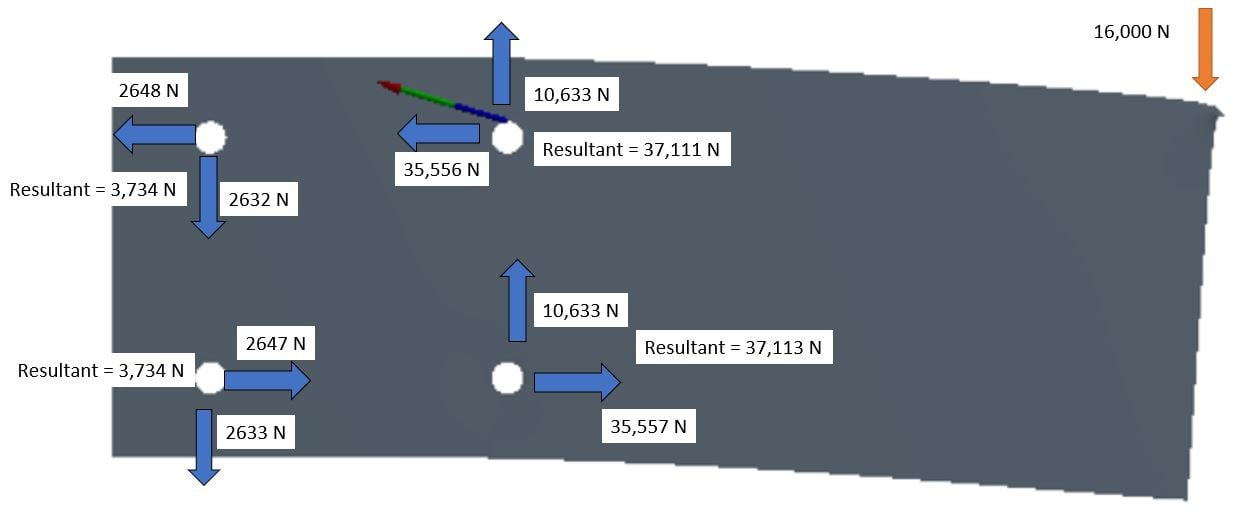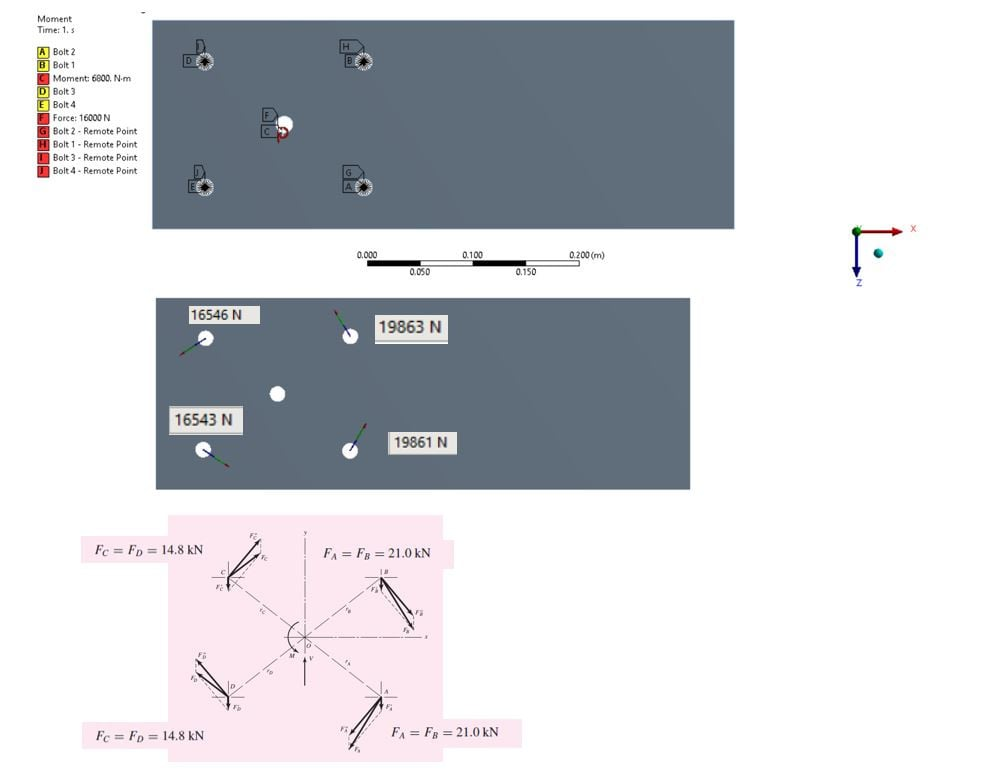## General Mechanical

Topics relate to Mechanical Enterprise, Motion, Additive Print and more

•Autonewbie
Subscriber

Hi I am wondering if I setup the ansys model wrongly as I get the results different from the hand calculation although both make sense to me...

In Ansys, I can calculate the forces accordingly... which I fixed four points... In hand calculation, I understand it is transferring the force from one point to a group of bolts... but I am a bit confused of the differences if I want to check how much forces are acting on the bolts...

•Rameez_ul_Haq
Subscriber
,so you are saying that the resultant forces are different for the hand calculations and the ANSYS results for each bolt? You haven't shown any resultant force on the ANSYS results.n
•Autonewbie
Subscriber
Ya... the resultant force not the same as calculated at the bolt...I upload picture again with resultant force...nn
•Rameez_ul_Haq
Subscriber
Did you model it properly? I mean the lengths of the plate, the location of the bolts and their diameters, location of the force, thickness of the plate? Try using a solid body instead of the plate and reconduct the analysis. Did you conduct the analysis using Large Deflection ON or OFF? If its OFF, try ON and vice versa.nThe directions of the force results in ANSYS seem correct though.n
•Autonewbie
Subscriber
Hi, I double check. All dimensions are correct.... It is about forces so, I guess large deflection not matter...n
•Rameez_ul_Haq
Subscriber
the distrbution of forces can change on the supports due to the non-linearity of geometry when Large Deflection is ON. Although the overall reaction force is going to be still equal to the external force.nMaybe there is a problem with the supports, I am guessing. Because the fixed supports are used to restrict all the DOF's of the nodes on the edge on which it is applied, and a restriction in a DOF of a node means that there needs to be a reaction force/moment there. Therefore, there can be some extra forces (in a different direction than in which the external force is applied) at each of the supports (not coming from the external force, but instead coming from the fixed nature of the support). These extra forces, when added to the ones you are getting from the book, might result in the overall force reactions you are seeing the ANSYS results. If you do a vector sum of all the forces you are seeing in the ANSYS, you will still get the same value as the externally applied force.nTry using a remote displacement on each of the bolts' edges, keep all the DOFs at constant 0 value, and keep the behavior as deformable. Check if its working.nIf not, try to use a different support.n
•Autonewbie
SubscriberI try to shift the force to the centroid of the bolts and add moment manually.. I can get pretty much the same forces... so it means FEA does not consider the moment?n
•Rameez_ul_Haq
Subscriber
,so what you are now doing is that you are pulling the centroid of all the supports by external force, and since all the supports are of the same diameter, the external force is going to distributed equally to all the supports, i.e. F/4. Since there is no external moment applied and it comes from the translation of the force to the centroid of the supports, the moment turns into a pair of equal forces since that is how the moment works [these equal forces coming from the moment will cancel each other out, and the net force is still going to be equal to external applied force]. nBut, in ANSYS, what I have experienced is that the first line of supports [which is relatively nearer to the applied force] is supposed to take bigger portion of forces than the line of supports which are relatively further from the externally applied load. But I think that the results given by the ANSYS makes more sense than the ones calculated from the theory because theory might have things more simplified.n
•Autonewbie
SubscriberI try to shift the force to the centroid of the bolts and add moment manually.. I can get pretty much the same forces... so it means FEA does not consider the moment?n
•Autonewbie
Subscriber
Yeah... you are right... I understand the one near to applied force would take up most of the forces ...n